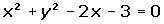Learn from home

The teachersChapters

To find the common points of a circle and a line, solve the systems formed by the equations of both.

In general, a quadratic equation is obtained, which will have a sign for the discriminant,, depending on the following solutions:## 1

Secant Line if Δ > 0

Two solutions: There are two points of intersection.## 2

Tangent line if Δ = 0

One solution: the line is a tangent to the circle.## 3

If Δ < 0

No solution: There is no intersection between the line and circle.

## Ejemplo

Calculate the points of intersection betweenand.Did you like the article?(1 votes, average: 5.00 out of 5)Loading...

Emma

I am passionate about travelling and currently live and work in Paris. I like to spend my time reading, gardening, running, learning languages and exploring new places.

Did you like
this resource?

Bravo!0 Followers

Most reacted commentRecent comment authors